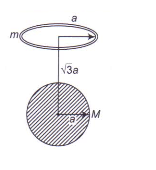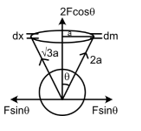Gravitational field intensity
Question

# A uniform ring of mass m is lying at a distance $\sqrt{3}$a form the centre of a sphere of mass M just over the sphere (where a is the radius of the ring as well as that of the sphere). Then magnitude of gravitational force between them isModerate
Solution

##For a small mass dm, distance from the center of sphere is 2a. force along ‘dm’  $\frac{\mathrm{G},\mathrm{M},\mathrm{dm}}{4{\mathrm{a}}^{2}}=\mathrm{F}$Component of force F sin   get cancelled net force 2Fcos $\mathrm{\theta }$ . For the entire ring- $\frac{\mathrm{GM}}{4{\mathrm{a}}^{2}}\frac{\mathrm{m}}{2}×2\mathrm{cos\theta }$$\frac{\mathrm{GMm}}{4{\mathrm{a}}^{2}}\frac{\sqrt{3}\mathrm{a}}{2\mathrm{a}}=\frac{\sqrt{3}\mathrm{GMm}}{8{\mathrm{a}}^{2}}$Alternative solution:-field intensity due the ring at the centre of the sphere is

Get Instant Solutions